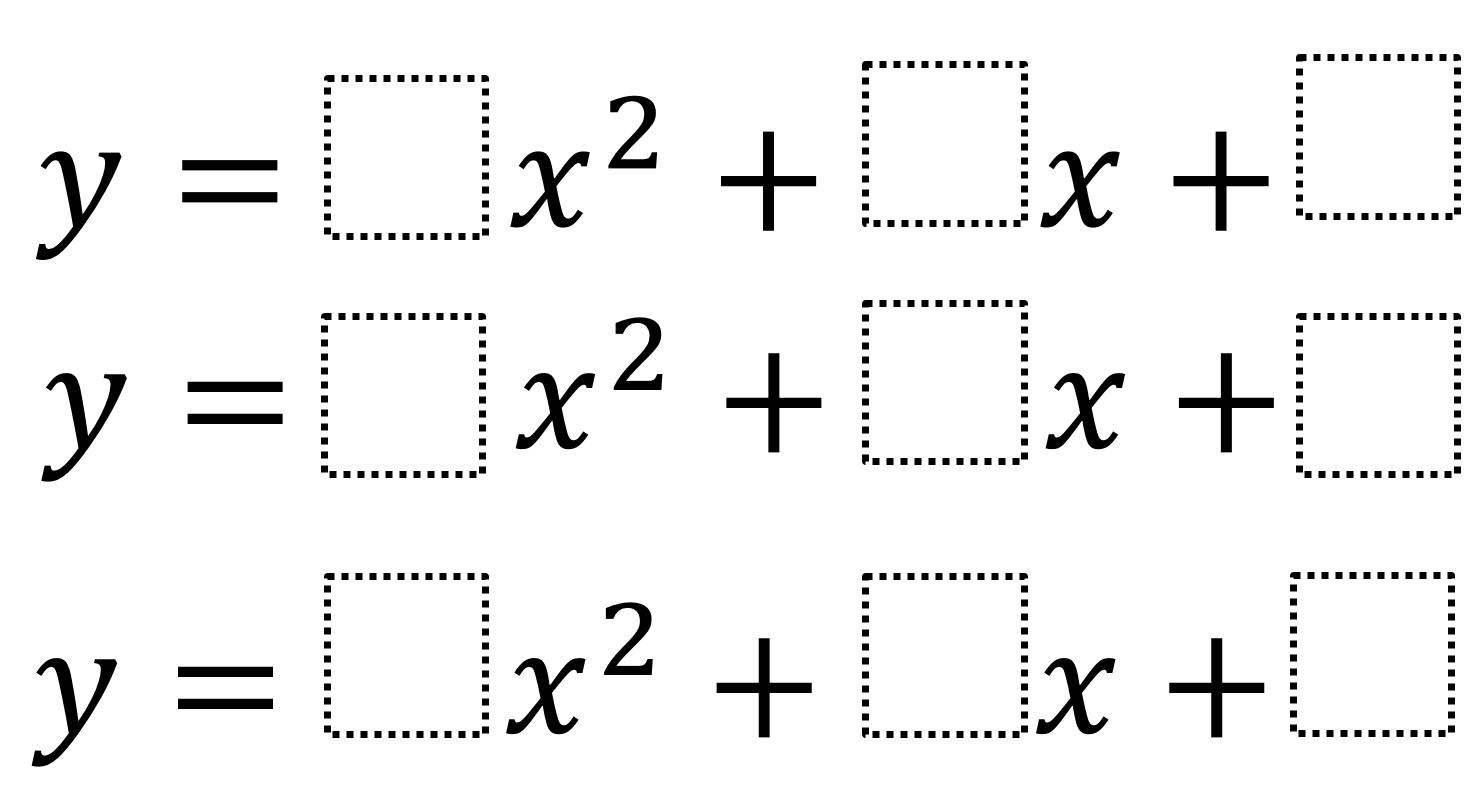Home > High School: Functions > Discriminant

# Discriminant

Directions: Using the whole numbers 0 through 9, no more than once, fill in the following boxes to make one function have no real roots, another function to have one real root and the last function have two real roots.### Hint

How do you know that a quadratic equation has only one solution?

There is more than one answer. Here are some possibilities:
a) y = 5x^2 + 3x + 7
b) y = 1x^2 + 6x + 9
c) y = 4x^2 + 8x + 2

Click on this link to see another possibility: Discriminant

Source: Lynda Chung

## Creating Sequences

Directions: Using the digits 0-9, at most one time each, complete the first three terms …